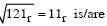Related Articles
GATE | GATE CS 2008 | Question 85
• Last Updated : 19 Nov, 2018

Let r denote number system radix. The only value(s) of r that satisfy the equation(A) decimal 10
(B) decimal 11
(C) decimal 10 and 11
(D) any value > 2

Answer: (D)

Explanation: As we can see 121 contains digit ‘2’ which can’t be represented directly in base ‘2’ (as digits should be less than base) so number system must have   “r>2”.

Ans (D) part.

Quiz of this QuestionMy Personal Notes arrow_drop_up